Chapter 11. Solutions

# Quantitative Units of Concentration

Learning Objectives

1. Learn to determine specific concentrations with several common units.

Rather than qualitative terms (see the later section “Some Definitions”), we need quantitative ways to express the amount of solute in a solution; that is, we need specific units of concentration. In this section, we will introduce several common and useful units of concentration.

Molarity (M) is defined as the number of moles of solute divided by the number of litres of solution: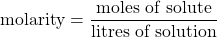which can be simplified as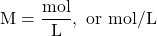As with any mathematical equation, if you know any two quantities, you can calculate the third, unknown, quantity.

For example, suppose you have 0.500 L of solution that has 0.24 mol of NaOH dissolved in it. The concentration of the solution can be calculated as follows: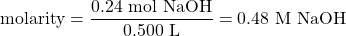The concentration of the solution is 0.48 M, which is spoken as “zero point forty-eight molarity” or “zero point forty-eight molar.” If the quantity of the solute is given in mass units, you must convert mass units to mole units before using the definition of molarity to calculate concentration. For example, what is the molar concentration of a solution of 22.4 g of HCl dissolved in 1.56 L? First, convert the mass of solute to moles using the molar mass of HCl (36.5 g/mol):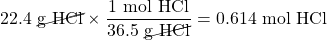Now we can use the definition of molarity to determine a concentration: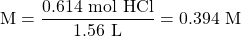Example 11.11

What is the molarity of a solution made when 32.7 g of NaOH are dissolved to make 445 mL of solution?

Solution
To use the definition of molarity, both quantities must be converted to the proper units. First, convert the volume units from millilitres to litres: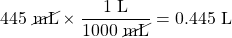Now we convert the amount of solute to moles, using the molar mass of NaOH, which is 40.0 g/mol: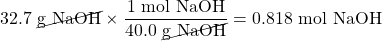Now we can use the definition of molarity to determine the molar concentration: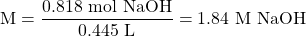Test Yourself
What is the molarity of a solution made when 66.2 g of C6H12O6 are dissolved to make 235 mL of solution?

1.57 M

The definition of molarity can be used to determine the amount of solute or the volume of solution, if the other information is given. Example 11.12 illustrates this situation.

Example 11.12

How many moles of solute are present in 0.108 L of a 0.887 M NaCl solution?

Solution

We know the volume and the molarity; we can use the definition of molarity to mathematically solve for the amount in moles. Substituting the quantities into the definition of molarity: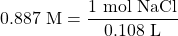We multiply the 0.108 L over to the other side of the equation and multiply the units together; “molarity × litres” equals moles, according to the definition of molarity. So: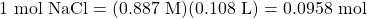Test Yourself
How many moles of solute are present in 225 mL of a 1.44 M CaCl2 solution?

0.324 mol

If you need to determine volume, remember the rule that the unknown quantity must be by itself and in the numerator to determine the correct answer. Thus rearrangement of the definition of molarity is required.

Example 11.13

What volume of a 2.33 M NaNO3 solution is needed to obtain 0.222 mol of solute?

Solution

Using the definition of molarity, we have: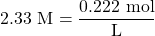To solve for the number of litres, we bring the 2.33 M over to the right into the denominator, and the number of litres over to the left in the numerator. We now have: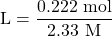Dividing, the volume is 0.0953 L = 95.3 mL.

Test Yourself

What volume of a 0.570 M K2SO4 solution is needed to obtain 0.872 mol of solute?

1.53 L

A similar unit of concentration is molality (m), which is defined as the number of moles of solute per kilogram of solvent, not per litre of solution: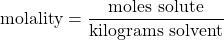Mathematical manipulation of molality is the same as with molarity.

Another way to specify an amount is percentage composition by mass (or mass percentage, % m/m). It is defined as follows: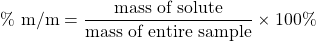It is not uncommon to see this unit used on commercial products.

Example 11.14

What is the mass percentage of Fe in a piece of metal with 87.9 g of Fe in a 113 g sample?

Solution
Using the definition of mass percentage, we have: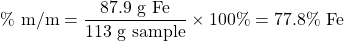Test Yourself
What is the mass percentage of H2O2 in a solution with 1.67 g of H2O2 in a 55.5 g sample?

3.01%

Related concentration units are parts per thousand (ppth), parts per million (ppm), and parts per billion (ppb). Parts per thousand is defined as follows: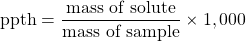There are similar definitions for parts per million and parts per billion: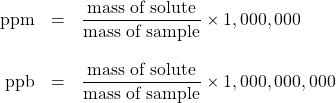Each unit is used for progressively lower and lower concentrations. The two masses must be expressed in the same unit of mass, so conversions may be necessary.

Example 11.15

If there is 0.6 g of Pb present in 277 g of solution, what is the Pb concentration in parts per thousand?

Solution
Use the definition of parts per thousand to determine the concentration. Substituting: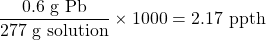Test Yourself
If there is 0.551 mg of As in 348 g of solution, what is the As concentration in ppm?

1.58 ppm

As with molarity and molality, algebraic rearrangements may be necessary to answer certain questions.

Example 11.16

The concentration of Cl ion in a sample of H2O is 15.0 ppm. What mass of Cl ion is present in 240.0 mL of H2O, which has a density of 1.00 g/mL?

Solution
First, use the density of H2O to determine the mass of the sample: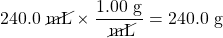Now we can use the definition of ppm: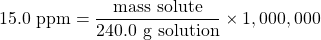Rearranging to solve for the mass of solute: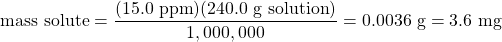Test Yourself
The concentration of Fe3+ ion in a sample of H2O is 335.0 ppm. What mass of Fe3+ ion is present in 3,450 mL of H2O, which has a density of 1.00 g/mL?

1.16 g

For ionic solutions, we need to differentiate between the concentration of the salt versus the concentration of each individual ion. Because the ions in ionic compounds go their own way when a compound is dissolved in a solution, the resulting concentration of the ion may be different from the concentration of the complete salt. For example, if 1 M NaCl were prepared, the solution could also be described as a solution of 1 M Na+(aq) and 1 M Cl(aq) because there is one Na+ ion and one Cl ion per formula unit of the salt. However, if the solution were 1 M CaCl2, there are two Cl(aq) ions for every formula unit dissolved, so the concentration of Cl(aq) would be 2 M, not 1 M.

In addition, the total ion concentration is the sum of the individual ion concentrations. Thus for the 1 M NaCl, the total ion concentration is 2 M; for the 1 M CaCl2, the total ion concentration is 3 M.

Key Takeaways

• Quantitative units of concentration include molarity, molality, mass percentage, parts per thousand, parts per million, and parts per billion.

Exercises

# Questions

1. Differentiate between molarity and molality.
2. Differentiate between mass percentage and parts per thousand.
3. What is the molarity of a solution made by dissolving 13.4 g of NaNO3 in 345 mL of solution?
4. What is the molarity of a solution made by dissolving 332 g of C6H12O6 in 4.66 L of solution?
5. How many moles of MgCl2 are present in 0.0331 L of a 2.55 M solution?
6. How many moles of NH4Br are present in 88.9 mL of a 0.228 M solution?
7. What volume of 0.556 M NaCl is needed to obtain 0.882 mol of NaCl?
8. What volume of 3.99 M H2SO4 is needed to obtain 4.61 mol of H2SO4?
9. What volume of 0.333 M Al(NO3)3 is needed to obtain 26.7 g of Al(NO3)3?
10. What volume of 1.772 M BaCl2 is needed to obtain 123 g of BaCl2?
11. What are the individual ion concentrations and the total ion concentration in 0.66 M Mg(NO3)2?
12. What are the individual ion concentrations and the total ion concentration in 1.04 M Al2(SO4)3?
13. If the C2H3O2 ion concentration in a solution is 0.554 M, what is the concentration of Ca(C2H3O2)2?
14. If the Cl ion concentration in a solution is 2.61 M, what is the concentration of FeCl3?

1. Molarity is moles per litre, whereas molality is moles per kilogram of solvent.
1. 0.457 M
1. 0.0844 mol
1. 1.59 L
1. 0.376 L
1. Mg2+ = 0.66 M; NO3 = 1.32 M; total: 1.98 M
1. 0.277 M